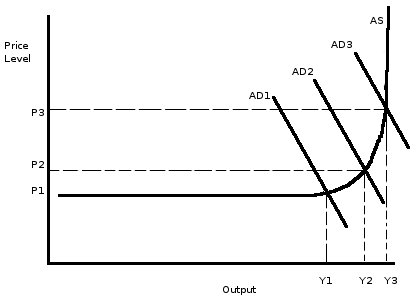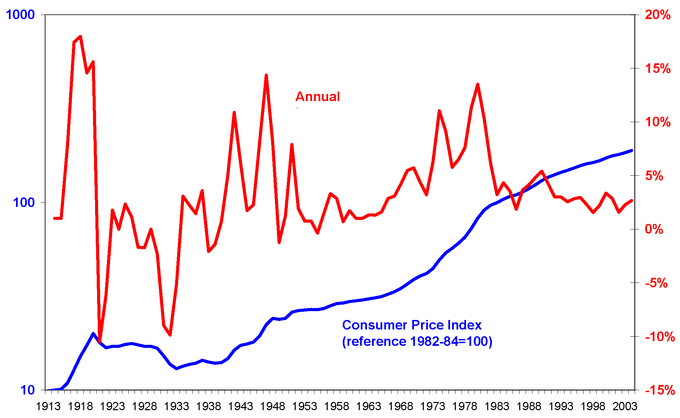## Introduction to Inflation

Inflation is a persistent increase in the general price level, and has three varieties: demand-pull, cost-push, and built-in.

### Learning Objectives

Distinguish between demand-pull and cost-push inflation

### Key Takeaways

#### Key Points

• Inflation is an increase in price levels, which decreases the real value, or purchasing power, of money.
• Demand -pull inflation is an increase in price levels due to an increase in aggregate demand when the employment level is full or close to full.
• Cost -push inflation is an increase in price levels due to a decrease in aggregate supply. Generally, this occurs due to supply shocks, or an increase in the price of production inputs.

#### Key Terms

• inflation: An increase in the general level of prices or in the cost of living.
• demand-pull inflation: A rise in the price level for goods and services in an economy due to greater demand than the economy’s ability to produce those goods and services.
• cost-push inflation: A rise in the price level for goods and services in an economy due to increases in the costs of production.

In economics, inflation is a persistent increase in the general price level of goods and services in an economy over a period of time. When the general price level rises, each unit of currency buys fewer goods and services. Consequently, inflation reflects a reduction in the purchasing power per unit of money; it is a loss of real value, as a single dollar is able to purchase fewer goods than it previously could.

### Types of Inflation

The reasons for inflation depend on supply and demand. Depending on the type of inflation, changes in either supply or demand can create an increase in the price level of goods and services. In Keynesian economics, there are three types of inflation.

### Demand-Pull Inflation

Demand-pull inflation is inflation that occurs when total demand for goods and services exceeds the economy’s capacity to produce those goods. Put another way, there is “too much money chasing too few goods. ” Typically, demand-pull inflation occurs when unemployment is low or falling. The increases in employment raise aggregate demand, which leads to increased hiring to expand the level of production. Eventually, production cannot keep pace with aggregate demand because of capacity constraints, so prices rise.Demand-Pull Inflation: Demand-pull inflation is caused by an increase in aggregate demand. As demand increases, so does the price level.

### Cost-Push Inflation

Cost-push inflation occurs when there is an increase in the costs of production. Unlike demand-pull inflation, cost-push inflation is not “too much money chasing too few goods,” but rather, a decrease in the supply of goods, which raises prices.Cost-Push Inflation: As the costs of production inputs rises, aggregate supply can decrease, which increases price levels.

The reason for decreases in supply are usually related to increases in the prices of inputs. One major reason for cost-push inflation are supply shocks. A supply shock is an event that suddenly changes the price of a commodity or service. (sudden supply decrease) will raise prices and shift the aggregate supply curve to the left. One historical example of this is the oil crisis of the 1970’s, when the price of oil in the U.S. surged. Because oil is integral to many industries, the price increase led to large increases in the costs of production, which translated to higher price levels.

### Built-In Inflation

Built-in inflation is the result of adaptive expectations. If workers expect there to be inflation, they will negotiate for wages increasing at or above the rate of inflation (so as to avoid losing purchasing power). Their employers then pass the higher labor costs on to customers through higher prices, which actually reflects inflation. Thus, there is a cycle of expectations and inflation driving one another.

## Defining and Calculating CPI

The consumer price index (CPI) is a statistical estimate of the change in prices of goods and services bought for consumption.

### Learning Objectives

Assess the uses and limitations of the Consumer Price Index

### Key Takeaways

#### Key Points

• The CPI is calculated by collecting the prices of a sample of representative items over a specific period of time.
• The CPI can be used to index the real value of wages, salaries, pensions, and price regulation. It is one of the most closely watched national economic statistics.
• The equation to calculate a price index using a single item is: $\text{Current CPI} = \text{Current item price} \times \text{Base year price} \times \frac{\text{Current CPI}}{\text{Base year CPI}}$.
• The equation for calculating the CPI for multiple items is: $\text{CPI for multiple items} = \frac{\text{Cost of CPI market basket at current period prices}}{\text{Cost of CPI market basket at base period prices}} \times 100.$.

#### Key Terms

• consumer price index: A statistical estimate of the level of prices of goods and services bought for consumption purposes by households.
• market basket: A list of items used specifically to track the progress of inflation in an economy or specific market.

### Consumer Price Index

The consumer price index (CPI) is a statistical estimate of the level of prices of goods and services bought for consumption by households. It measures changes in the price level of a market basket of goods and services used by households. The CPI is calculated by collecting the prices of a sample of representative items over a specific period of time. Goods and services are divided into categories, sub categories, and sub indexes. All of the information is combined to produce the overall index of consumer expenditures. The annual percentage change in a CPI is used to measure inflation. The CPI can be used to index the real value of wages, salaries, pensions, and price regulation. It is one of the most closely watched national economic statistics.Consumer Price Index: The graph shows the consumer price index in the United States from 1913 – 2004. The x-axis indicates year, the left y-axis indicates the Consumer Price Index, and the right y-axis indicates annual percentage change in Consumer Price Index, which can be used to measure inflation.

### Calculating CPI using a Single Item

In order to calculate the CPI using a single item the following equation is used:

$\text{Current CPI} = \text{Current item price} \times \text{Base year price} \times \frac{\text{Current CPI}}{\text{Base year CPI}}$

### Calculating the CPI for Multiple Items

When calculating the CPI for multiple items, it must be noted that many but not all price indices are weighted averages using weights that sum to 1 or 100. When calculating the average for a large number of products, the price is given a weighted average between 1 and 100 to simplify calculation. The weighting determines the importance of the quantity of the product on average. The equation for calculating the CPI for multiple items is:

$\text{CPI for multiple items} = \frac{\text{Cost of CPI market basket at current period prices}}{\text{Cost of CPI market basket at base period prices}} \times 100.$

For example, imagine you buy five sandwiches, two magazines, and two pairs of jeans. In the first period, sandwiches are $6 each, magazines are$4 each, and jeans are $35 each. This will be our base period. In the second period, sandwiches are$7, magazines are $6, and jeans are$45.

Market basket at base period prices = 5(6.00) + 2(4.00) + 2(35.00) = 108.00.

Market basket at current period prices = 5(7.00) + 2(6.00) + 2(45.00) = 137.00.

$\text{CPI for multiple items} = \frac{137}{108} \times 100 = 127$

The CPI based on consumption is 127.

### CPI Limitations

The CPI is a convenient way to calculate the cost of living and price level for a certain period of time. However, the CPI does not provide a completely accurate estimate for the cost of living. Issues that impede the accuracy of the CPI include substitution bias (consumers substituting goods for others), introducing new products, and changes in quality. The CPI can also overstate inflation because it does not always account for quality improvements or new goods and services.

### GDP Deflator vs. CPI

The GDP deflator is a measure of the level of prices of all new, domestically produced, final goods and services in an economy. Unlike the CPI, the GDP deflator is a measure of price inflation or deflation for a specific base year. The GDP deflator differs from the CPI because it is not based on a fixed basket of goods and services. The GDP deflator “basket” changes from year to year depending on people’s consumption and investment patterns. Unlike the CPI, the GDP deflator is not impacted by substitution biases. Despite the GDP being more flexible, the CPI is a more accurate reflection of the changes in the cost of living.